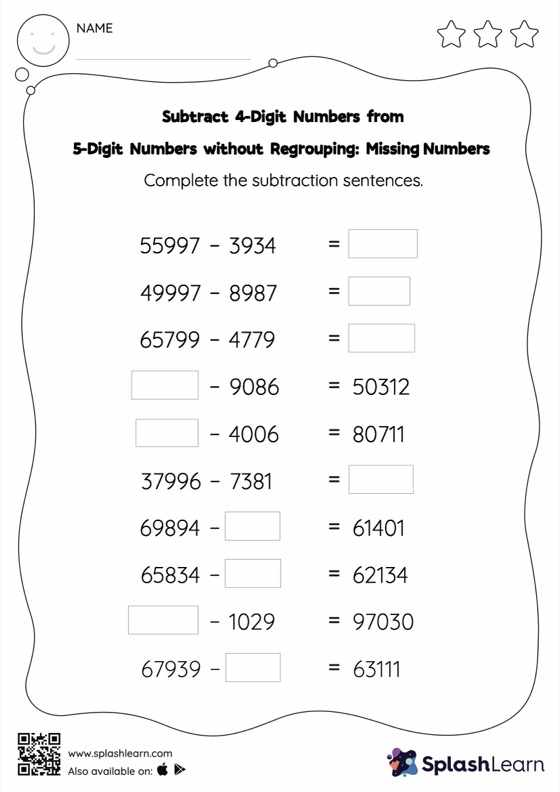# Subtract 4-Digit Numbers from 5-Digit Numbers without Regrouping: Missing Numbers Worksheet

Home > Subtract 4-Digit Numbers from 5-Digit Numbers without Regrouping: Missing NumbersCan your young mathematician subtract 4-digit numbers from 5-digit numbers without regrouping? That's exactly what they do here. In this worksheet, students practice solving problems written in the horizontal format. How numbers are laid out in a problem affects the method a student employs to solve it. Therefore to develop actual fluency and mastery of multiple strategies, students must practice different formats.